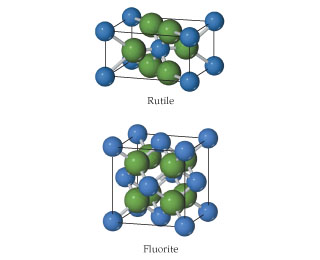# Problem: The rutile and fluorite structures, shown here (anions are colored green and are the larger of the two spheres), are two of the most common structure types for ionic compounds where the cation-to-anion ratio is 1:2.For CaF2 and ZnF2 use ionic radii, Ca2+ (r=1.14), Zn2+ (r=0.88), and F- (r=1.19), to predict which compound is more likely to crystallize with the fluorite structure and which with the rutile structure.

⚠️Our tutors found the solution shown to be helpful for the problem you're searching for. We don't have the exact solution yet.

###### Problem Details

The rutile and fluorite structures, shown here (anions are colored green and are the larger of the two spheres), are two of the most common structure types for ionic compounds where the cation-to-anion ratio is 1:2.For CaF2 and ZnF2 use ionic radii, Ca2+ (r=1.14), Zn2+ (r=0.88), and F- (r=1.19), to predict which compound is more likely to crystallize with the fluorite structure and which with the rutile structure.